## Practical Geometry Exercise 14.1 Solutions Ncert class 10

Practical Geometry Exercise 14.1 Solutions Ncert Class 6th textbook are given.

First you should study the textbook lesson Practical Geometry very well.

You should also observe the example problems and solutions given in the textbook.

Observe the given below solutions and try them in your own method.

Chapter 1 Knowing our Numbers

Chapter 2 whole Numbers

Some chapters solutions links are given below.

M

### Exercise 14.1 Practical Geometry Solutions Ncert class 10

Chapter – 14  Practical Geometry Exercise 14.1 Solutions Class 6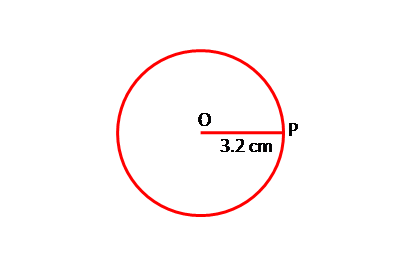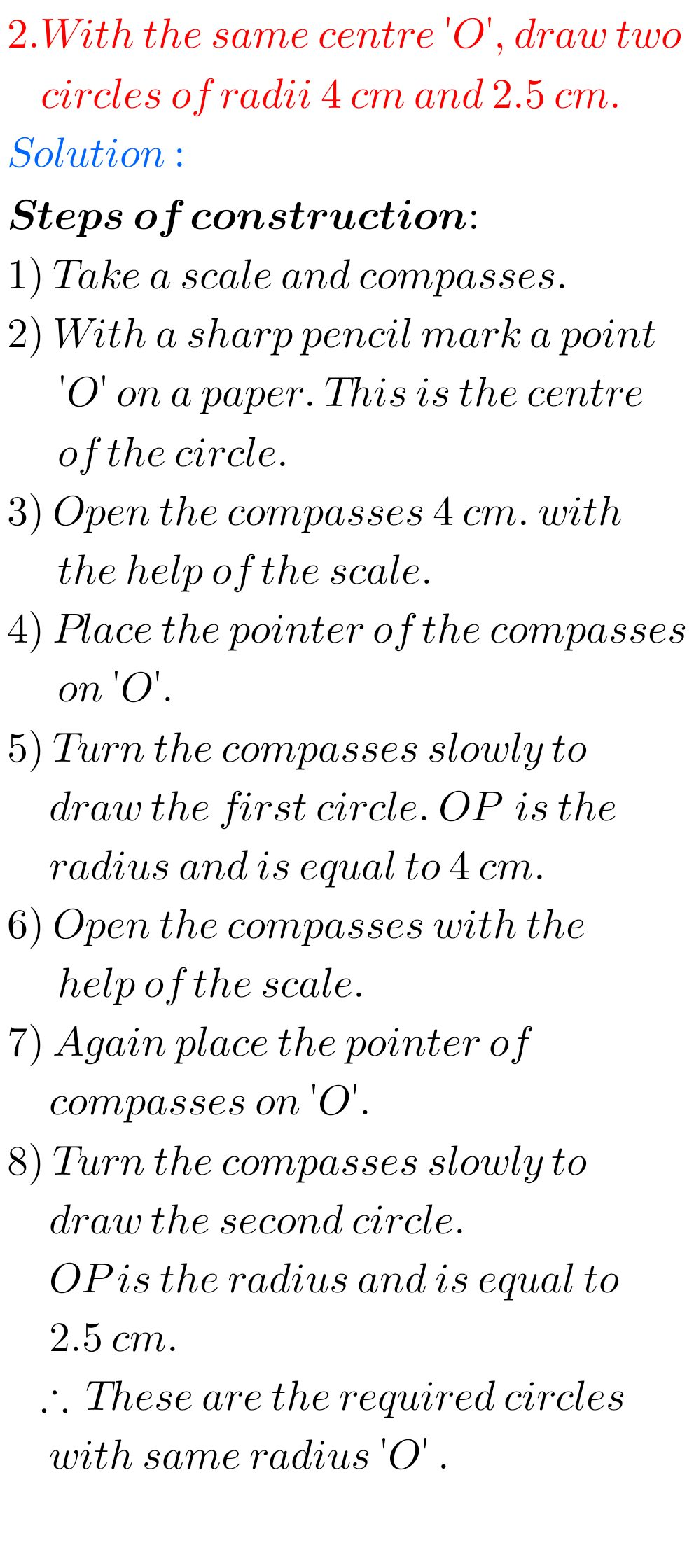#### Solutions for class 6 Practical Geometry Ncert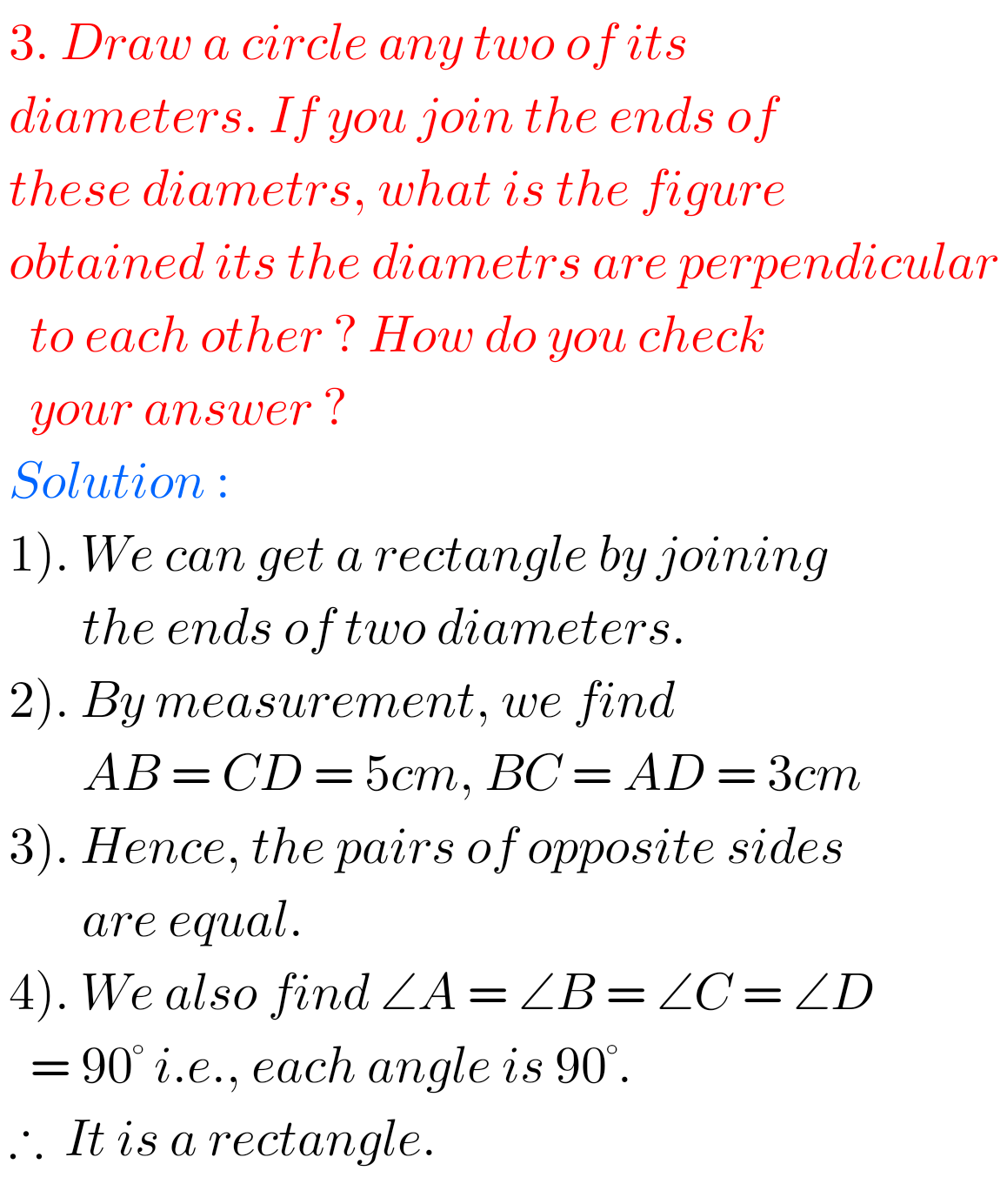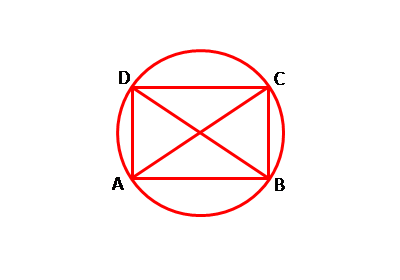##### 6th class Practical Geometry Solutions Ncert Exercise 14.1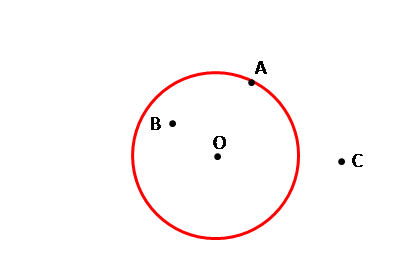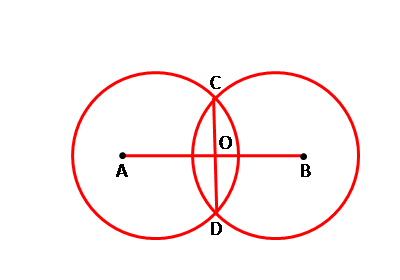Note : Observe the solutions and try them in your own method.

Chapter 3 Playing with Numbers

Chapter 4 Basic Geometrical Ideas

Exercise 4.1

Exercise 4.2

Exercise 4.3

Exercise 4.4

Exercise 4.5

Exercise 4.6

Integers

Chapter 6 Integers

Fractions

Chapter 14 Practical Geometry

Exercise 14.1

Exercise 14.2

Exercise 14.3

Exercise 14.4

Exercise 14.5

Exercise 14.6